System ProgrammingLearn Decision Making using Switch Statement in C++

# Learn Decision Making using Switch Statement in C++

After discussing the decision making using if statement with its various forms, we are now on a date with another useful decision making tool: the switch statement. The switch statement is useful when we have a long list of possible options (values) to be tested against only one variable.

To imagine the problem, let’s go back to the menu example. The program displays a menu of options, and asks the user to enter his choice. The choice is typically an integer number (or sometimes a letter). Once entered and validated, the user’s choice is compared against the list of available options to determine what actions to be taken (i.e. what code to be executed). In the simple menu example discussed in the previous article, the matter was really simple; the menu offered the user only three options (1, 2, and 99)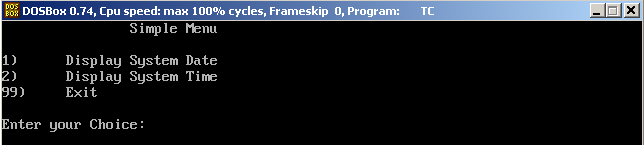What if the menu was longer?! What if it contains more options that just displaying system date and time?! Consider the case wherein the menu should also offer displaying host name, IP address(es), and also offers changing them all?! There are eight options, besides to the exit option = nice different choices available. Now, the hard part is: how to code this?!

Obviously, we need a series of if .. else if  statements to decide (based on the user’s input) what the program will do. This will be something like the following:

```If(choice==1)
{
// display system date
…
}
else if(choice==2)
{
//
…
}

…

else if (choice==8)
{
// Execute something
…
}
else if (choice==99)
{
// exit the program
…
}
else
{
//the user has entered an invalid choice
}
```

The checks are made for different values against the same variable. Each possible offered option required a separate check, resulting in nice checks. Now, what if the menu was even longer?! The switch statement offers a more efficient way to implement this requirement.

Syntax

```switch (x)
{
case x1:
statement1;
statement2;
…
statementn;
break;
case x2:
statement1;
statement2;
…
statementn;
break;

…

case xn:
statement1;
statement2;
…
statementn;
break;
default:
statement1;
statement2;
…
statementn;
}
```

Where: x1, x2, … , xn are the possible values to check the variable x against.
break is a C++ statement that exits the switch block.
default  is a C++ keyword that acts as the last resort if neither of the case values had a match. Does this look familiar?! Yes, exactly!! The else statement job in the if .. else if .. else structure. They both do the same job.

Example
Consider the basic calculator example. In this new version, we are going to prompt the user to enter the operation he wants to perform using the format: X operator Y

Where X and Y are the operands, and the operator is either +, -, *, or /
The program should make the required calculation and print the result to the user.

Consider the following code:

```#include<iostream.h>
#include<conio.h>
void main()
{
float x,y,result;
char op;
clrscr();
cout<<"Enter the operation you want to perform in the format:\n";
cout<<"\tX op Y ( e.g. 5 + 8 ): ";
cin >> x >> op >> y;
switch (op)
{
case '+':
result = x + y; 	break;
case '-':
result = x - y;     	break;
case '*':
result = x * y;		break;
case '/':
result = x / y;		break;
}
cout << endl << x << " " << op << " " << y
<< " = " << result;
getch();
}
```

Let’s see how this program will behave: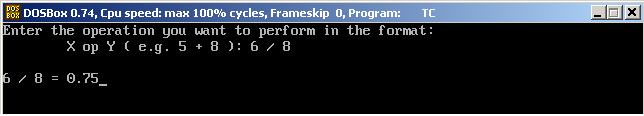And with subtraction: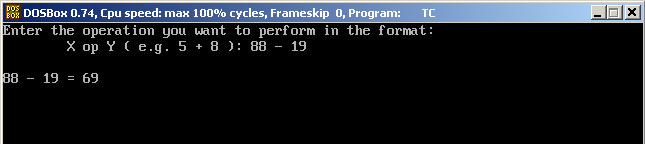And one more time with multiplication: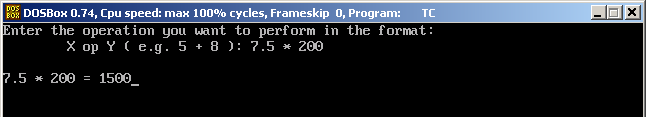Great!! Now, several things need some explanation:

• char op;

This statement declares a variable op of type char (character).

• The statement: cin >> x >> op >> y;

prompts the user to type three inputs of two different types (float and char).

• The final statement that displays the result to the user:
```cout << endl << x << " " << op << " " << y
<< " = " << result;
```

was written in two lines. cin and cout statements can span multiple lines as desired.

In think the rest of the code is simple enough to be easily understood.Conditional Operator
Consider the following code:

```if (CONDITION)
var = something;
else
var = something_else;
```

The Conditional Operator presents a very compact alternative to the above code:

`var  = (CONDITION) ? something : something_else;`

I think the line is self-explanatory, so I prefer to illustrate it by an example.

Example
In this example, we are going to re-write the Odd-Even program using the conditional operator.

Consider the following code.

```#include <iostream.h>
#include <conio.h>
void main()
{
int num;
cout << "\nEnter an Integer number: ";
cin >> num;
cout << endl << num << " is ";
(num % 2 == 0) ? cout << "Even" : cout << "Odd";
cout << endl;
getch();
}
```

Let’s execute it.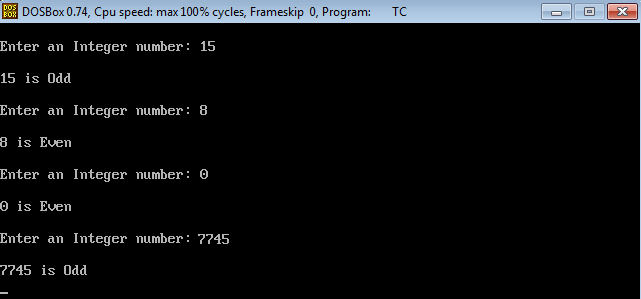Perfect!!

* * * * * *
Summary

• The switch statement can replace the if..else if structure in case different numeric values or characters are being tested against the same variable.
• The conditional operator could be a compact alternative to a simple if statement with a condition and single-statement body, and else part having also a single-statement body.

That was the topic of Decision Making. In the next article, we are going to talk about Loops. An interesting topic that you shouldn’t miss. Waiting for you there!!!

### Build A Front End Of an e-Commerce Site Using React

21,501Fans
4,213Followers
104,000Subscribers

### Build A Front End Of an e-Commerce Site Using React

- Advertisement -